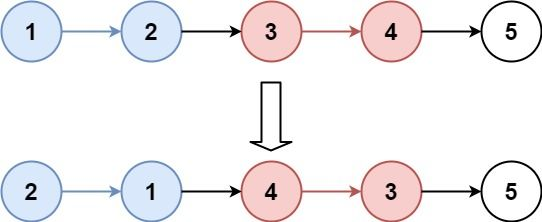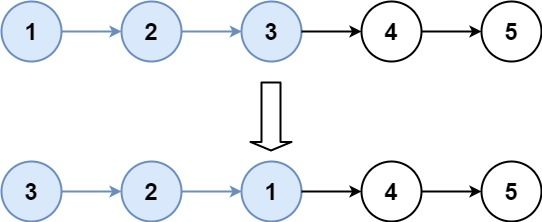# Question

Formatted question description: https://leetcode.ca/all/25.html

Given the head of a linked list, reverse the nodes of the list k at a time, and return the modified list.

k is a positive integer and is less than or equal to the length of the linked list. If the number of nodes is not a multiple of k then left-out nodes, in the end, should remain as it is.

You may not alter the values in the list's nodes, only nodes themselves may be changed.

Example 1:Input: head = [1,2,3,4,5], k = 2
Output: [2,1,4,3,5]


Example 2:Input: head = [1,2,3,4,5], k = 3
Output: [3,2,1,4,5]


Constraints:

• The number of nodes in the list is n.
• 1 <= k <= n <= 5000
• 0 <= Node.val <= 1000

Follow-up: Can you solve the problem in O(1) extra memory space?

# Algorithm

To reverse a linked list in groups of size k, we first need to divide the original linked list into several small segments, each containing k nodes, and then flip each segment separately. This requires two functions, one for segmentation and one for flipping.

For example, consider the linked list 1->2->3->4->5. When dealing with linked list problems, it’s common to add a dummy node at the beginning because the head node may change when the linked list is flipped. To keep track of the current latest position of the head node, we introduce a dummy node before the original head node. In this case, the linked list after adding the dummy node becomes -1->1->2->3->4->5.

To reverse the group of nodes 1, 2, and 3 when k is 3, we need two pointers: pre and next. pre points to the node before the group to be flipped, and next points to the node after the group to be flipped. After flipping the group, the position of pre is updated to the following new position.

prev is dummy, then current is always node ‘1’, ‘1’ will move each iteration of while loop for k-group swap

prev is static during the full while loop of while (kcopy > 0)

1->2->3->4->5 , k=3

2,1,3,4,5
3,2,1,4,5
=> always getting 1's next for prev's next => current (below) not changing in one-batch-swap


# Code

• public class Reverse_Nodes_in_k_Group {

class Solution {
public ListNode reverseKGroup(ListNode head, int k) {
ListNode dummy = new ListNode(0);

ListNode prev = dummy;

// count total nodes
int count = 0;
while (tmp != null) {
count++;
tmp = tmp.next;
}

// 1->2->3->4->5 , k=3
// 2,1,3,4,5
// 3,2,1,4,5
// => always getting 1's next for prev's next => current (below) not changing in one-batch-swap

// if only one node left, then no swap
while (count >= k) {

ListNode originalFirst = prev.next;

int kcopy = k - 1; // @note: since current node is already counted as 1
while (kcopy > 0) { // both prev and current, not changed in while loop

ListNode nextNextCopy = originalFirst.next.next;
ListNode firstInGroup = prev.next;

prev.next = originalFirst.next;
prev.next.next = firstInGroup;
originalFirst.next = nextNextCopy;

kcopy--;
}

// @note: update previous AND current. I forgot current...
prev = originalFirst; // now current is the last one of this group
count -= k;
}

return dummy.next;
}
}
}


• // OJ: https://leetcode.com/problems/reverse-nodes-in-k-group/
// Time: O(N)
// Space: O(1)
class Solution {
public:
ListNode* reverseKGroup(ListNode* head, int k) {
ListNode h, *tail = &h;
auto prev = tail;
int i = 0;
for (auto p = head; i < k && p; ++i, p = p->next);
if (i < k) {
break;
}
for (int i = 0; i < k && head; ++i) {
node->next = prev->next;
prev->next = node;
}
while (tail->next) tail = tail->next;
}
return h.next;
}
};

• # Definition for singly-linked list.
# class ListNode:
#     def __init__(self, val=0, next=None):
#         self.val = val
#         self.next = next
class Solution:
def reverseKGroup(self, head: ListNode, k: int) -> ListNode:
while p:
q = p.next
p.next = pre
pre = p
p = q
return pre

pre = cur = dummy
while cur.next:
for _ in range(k):
cur = cur.next
if cur is None:
return dummy.next
t = cur.next
cur.next = None # cut from next k-group, so to reverseList() for current k-group
start = pre.next
pre.next = reverseList(start)
start.next = t # so now 'start' is the last node of k-group
pre = cur = start # same as the reset before while loop
return dummy.next

############

# class ListNode(object):
#     def __init__(self, x):
#         self.val = x
#         self.next = None

class Solution(object):
"""
:type k: int
:rtype: ListNode
"""

pre = None
while cur and k > 0:
tmp = cur.next
cur.next = pre
pre = cur
cur = tmp
k -= 1
return cur, pre

length = 0
while p:
length += 1
p = p.next
if length < k:
step = length / k
ret = None
pre = None
while p and step:
if ret is None:
if pre:
pre = p
p = next
step -= 1
return ret


• /**
* type ListNode struct {
*     Val int
*     Next *ListNode
* }
*/
func reverseKGroup(head *ListNode, k int) *ListNode {
for i := 0; i < k; i++ {
if end == nil {
}
end = end.Next
}
res := reverse(start, end)
start.Next = reverseKGroup(end, k)
return res
}

func reverse(start, end *ListNode) *ListNode {
var pre *ListNode = nil
for start != end {
tmp := start.Next
start.Next, pre = pre, start
start = tmp
}
return pre
}


• /**
* class ListNode {
*     val: number
*     next: ListNode | null
*     constructor(val?: number, next?: ListNode | null) {
*         this.val = (val===undefined ? 0 : val)
*         this.next = (next===undefined ? null : next)
*     }
* }
*/

function reverseKGroup(head: ListNode | null, k: number): ListNode | null {
let dummy = new ListNode(0, head);
let pre = dummy;
let tail = pre;
for (let i = 0; i < k; ++i) {
tail = tail.next;
if (tail == null) {
return dummy.next;
}
}
let t = tail.next;
// set next
tail.next = t;
// set new pre and new head
pre = tail;
}
return dummy.next;
}

function reverse(head: ListNode, tail: ListNode) {
let pre = tail.next;
// head -> next -> ... -> tail -> pre
while (pre != tail) {
let t = cur.next;
cur.next = pre;
pre = cur;
cur = t;
}
}


• /**
* public class ListNode {
*     public int val;
*     public ListNode next;
*     public ListNode(int val=0, ListNode next=null) {
*         this.val = val;
*         this.next = next;
*     }
* }
*/
public class Solution {
public ListNode ReverseKGroup(ListNode head, int k) {
ListNode dummy = new ListNode(0, head);
ListNode pre = dummy, cur = dummy;
while (cur.next != null)
{
for (int i = 0; i < k && cur != null; ++i)
{
cur = cur.next;
}
if (cur == null)
{
return dummy.next;
}
ListNode t = cur.next;
cur.next = null;
ListNode start = pre.next;
pre.next = ReverseList(start);
start.next = t;
pre = start;
cur = pre;
}
return dummy.next;
}

ListNode pre = null, p = head;
while (p != null)
{
ListNode q = p.next;
p.next = pre;
pre = p;
p = q;
}
return pre;
}
}